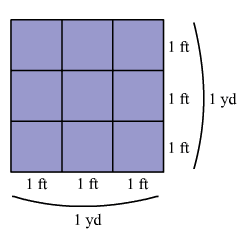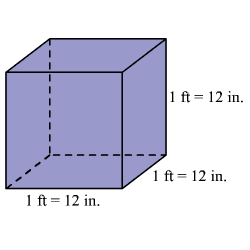# Convert Units of Area and Volume

The objective of the lesson is to convert units of measure between dimensions including area and volume .

### Area:

The area is measured in square units (unit $2$ ).

Example 1:

Convert one square yard to square feet.A square yard is a square with a side length of one yard.

We know that, $1$ yard = $3$ feet.

So, $1$ square yard is a square with side length $3$ feet.

You can use the formula for the area of a square, $A={s}^{2}$ , to convert square units.

Since $1$ yard = $3$ feet, substitute $s$ by $3$ .

$\begin{array}{l}A={\left(3\right)}^{2}\\ \text{\hspace{0.17em}}\text{\hspace{0.17em}}\text{\hspace{0.17em}}\text{\hspace{0.17em}}\text{\hspace{0.17em}}=9\end{array}$

So, $1$ square yard is equal to $9$ square feet.

### Common conversions for Square Units

The table gives several common measurement conversions for square units.

 Customary Units Metric Units

### Volume

The volume is measured in cubic units (unit $3$ ).

Example 2:

Convert one cubic foot to cubic inches.A cubic foot is a cube with a side length of one foot.

We know that, $1$ foot = $12$ inches.

So, $1$ cubic foot is a cube with side length $12$ inches.

You can use the formula for the volume of a prism, $V=bwh$ , to convert cubic units.

Since $1$ foot = $12$ inches, replace $b$ , $w$ , and $h$ with $12$ .

$\begin{array}{l}V=\left(12\right)\left(12\right)\left(12\right)\\ \text{\hspace{0.17em}}\text{\hspace{0.17em}}\text{\hspace{0.17em}}\text{\hspace{0.17em}}\text{\hspace{0.17em}}=1,728\end{array}$

So, $1$ cubic foot is equal to $1,728$ cubic inches.

### Common conversions for cubic units

The table gives several common measurement conversions for cubic units.

 Customary Units Metric Units

The metric system also relates length, mass, and capacity.

$1$ milliliter has the same volume as $1$ cubic centimeter. ( $1$ mL = $1$ cc)

$1$ milliliter of water is approximately $1$ gram. ( )

Note:

A length is measured in units , area is measured in square units $\left(\text{unit}×\text{unit}={\text{unit}}^{2}\right)$ , and volume is measured in cubic units $\left(\text{unit}×\text{unit}×\text{unit}={\text{unit}}^{3}\right)$ .